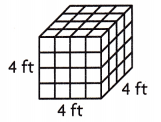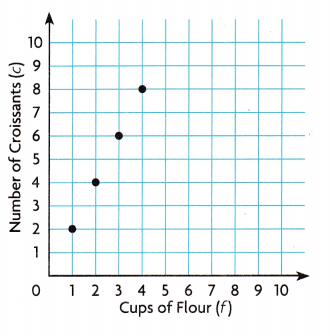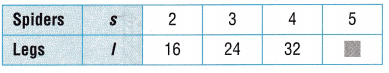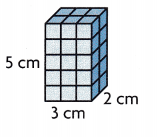Refer to our Texas Go Math Grade 5 Answer Key Pdf to score good marks in the exams. Test yourself by practicing the problems from Texas Go Math Grade 5 Unit 3 Assessment Answer Key.

Vocabulary

Choose the best term from the box.

Vocabulary
composite number
cubic units
formula
prime number

Question 1.
A ___________ has more than two factors. (p. 291)
Answer: A composite number has more than two factors.

Question 2.
Volume is measured using __________. (p. 355)
Answer: Volume is measured using cubic units.

Concepts and Skills

List all the factor pairs for the number. Tell whether the number is prime or composite. (TEKS 5.4.A)

Texas Go Math Grade 5 Unit 3 Assessment Answer Key Question 3.
69
The factor pairs of 69 are:
1 × 69 = 69
3 × 23 = 69
Thus it is a composite number.

Question 4.
17
1 × 17 = 17
17 × 1 = 17
Thus 17 is a multiple of one and itself.
Thus it is a prime number.

Question 5.
63
The factor pairs of 63 are:
1 × 63 = 63
3 × 21 = 63
7 × 9 = 63
Thus it is a composite number.

Simplify the numerical expression. (TEKS 5.4.F)

Question 6.
35 – (9 + 6)
35 – (9 + 6)
35 – (15) = 35 – 15 = 20

Question 7.
15 + (20 – 4) + 4
15 + (20 – 4) + 4
15 + 16 + 4
= 15 + 20
= 35

Question 8.
6 × [(5 × 3) – (2 + 9)]
6 × [(5 × 3) – (2 + 9)]
6 × [15 – 11]
6 × 4 = 24

Go Math Expressions Grade 5 Unit 3 Test Answer Key Question 9.
Use formulas to find the area and perimeter. (TEKS 5.4.H)Perimeter: _________________
Area: ________________
The above figure is a rectangle.
length = 5 cm
width = 6 cm
We know that,
area of the rectangle = lw
A = 5 × 6 = 30 sq.cm
perimeter of the rectangle = 2l + 2w
P = 2 (l + w)
P = 2 (5 + 6)
P = 2(11) = 22 cm
Perimeter: 22 cm
Area: 30 sq.cm

Question 10.
Use a formula to find the volume. (TEKS 5.4.G)Volume: ________________
Volume of a cube = 4 ft × 4 ft × 4 ft
V = 64 cu. ft

Question 11.
Moesha has some books. Her mother gives her 5 new books and her father gave her 4 new books. Now Moesha has 11 books. If s stands for the number of books Moesha starts with, which equation represents the problem? (TEKS5.4.B)
(A) s = 11 + 5
(B) 11 = 5 × 4 + s
(C) s = 11 × 5 + 4
(D) 11 = s + 5 + 4
Answer: 11 = s + 5 + 4
The correct answer is option D.

Texas Go Math Grade 5 Pdf Unit 3 Assessment Answer Key Question 12.
The graph shows the amount of flour it takes to make croissants. Which rule describes the pattern on the graph? (TEKS 5.4.D)(A) c = f + 1
(B) f = c + 1
(C) c = 2f
(D) f = 2c

Question 13.
Marcello needs to cut a piece of canvas that is 48 sq cm. The width of the sheet of canvas is 8 cm. Which of the following shows the equation Marcello could have used and the length of the canvas? (TEKS 5.4.B, 5.4.H)
(A) 64 sq cm, l = 8 × 8
(B) 6 cm, 48 = 8 × l
(C) 32 cm, 8 + 8 + 8 + 8 = l
(D) 12 cm, 48 = 4 × l
Given,
Marcello needs to cut a piece of canvas that is 48 sq cm.
The width of the sheet of the canvas is 8 cm.
(B) 6 cm, 48 = 8 × l

Question 14.
Steve uses the rule l = 8s to determine the number of legs 5 spiders have. What is the value of ☐? (TEKS 5.4.C)(A) 8
(B) 40
(C) 10
(D) 14
5 × 8 = 40
Thus the correct answer is option B.

Go Math Expressions Grade 5 Answer Key Pdf Unit 3 Question 15.
An adult elephant eats about 300 pounds of food each day. Which expression shows about how many pounds of food 12 elephants eat in 5 days? (TEKS 5.4.E)
(A) 5 + (300 × 12)
(B) 5 × (300 × 12)
(C) (300 × 12) ÷ 5
(D) (300 × 12) – 5
Given,
5 × (300 × 12)
Thus the correct answer is option B.

Question 16.
Paula is building a box to store her marble collection. The box is a rectangular prism. The area of the base will be 15 sq inches. She wants the volume of the box to be 150 cu in. Which of the following shows the equation Paula can use and the height of the box? (TEKS 5.4.B, 5.4.H)
(A) 150 = 15 h, 10 in.
(B) h + 15 = 150, 135 in.
(C) h = 15 × 15, 225 sq in.
(D) 150 = h × 15, 5 in.
Given,
Paula is building a box to store her marble collection. The box is a rectangular prism.
The area of the base will be 15 sq inches. She wants the volume of the box to be 150 cu in.
150 = 15 h, 10 in.
Thus the correct answer is option A.

Question 17.
Ling uses a formula to find the area of her rectangular garden. The garden measures 12 feet by 5 feet. She wants to divide the garden into 10 equal areas. Which equation can Ling use to find out how large each area, t, will be? (TEKS 5.4.B, 5.4.H)
(A) (12 × 5) ÷ t = 10
(B) t = 12 × 5 × 10
(C) t = (12 + 5) ÷10
(D) (12 × 5) ÷ 10 = t
Given,
The garden measures 12 feet by 5 feet. She wants to divide the garden into 10 equal areas.
(12 × 5) ÷ t = 10

Question 18.
Amy builds a rectangular prism using centimeter cubes. The length of the prism is 3 centimeters. The width is 2 centimeters and the height is 5 centimeters. Which equation shows a formula for finding the volume of the rectangular prism? (TEKS 5.4.B, 5.4.G)(A) V = 3 × 2
(B) V = 3 × 5
(C) V = 6 × 5
(D) V = 3 + 2 + 5
Given,
Amy builds a rectangular prism using centimeter cubes.
The length of the prism is 3 centimeters. The width is 2 centimeters and the height is 5 centimeters.
V = 6 × 5
Thus the correct answer is option C.

A pack of 8 bottles of olive oil costs $96. Marsha wants to write an equation to calculate the cost of 1 bottle. If b represents the cost of 1 bottle of olive oil, which of the following shows the equation Marsha could use and the cost of 1 bottle? (TEKS 5.4.B) (A) 96 = b + 8,$88
(B) 96 = 8b, $12 (C) Not here (D) b = 96 ÷ 8,$16
Answer:96 = b + 8, $88 Question 21. Which equation describes the pattern in the table below? (TEKS 5.4.D)(A) c = w × 3 (B) c = w + 4 (C) c = w × 4 (D) w = c × 3 Answer: (A) c = w × 3 5th Grade Unit 3 Math Unit 3 End of Unit Assessment Question 22. Drew’s weekly allowance is$8. Jan’s weekly allowance is $10. Drew spends$3 a week. Jan spends $4 a week. The video game Drew and Jan want to buy costs$55. Write an expression to show how many weeks it will take them to save $55. Then simplify the expression. Describe the steps you used to write and simplify the expression. (TEKS 5.4.E, 5.4.F) Answer: Drew has 8 and spends 3 so ($8-3$) Jan has 10 and spends 4 so ($10-\$4)